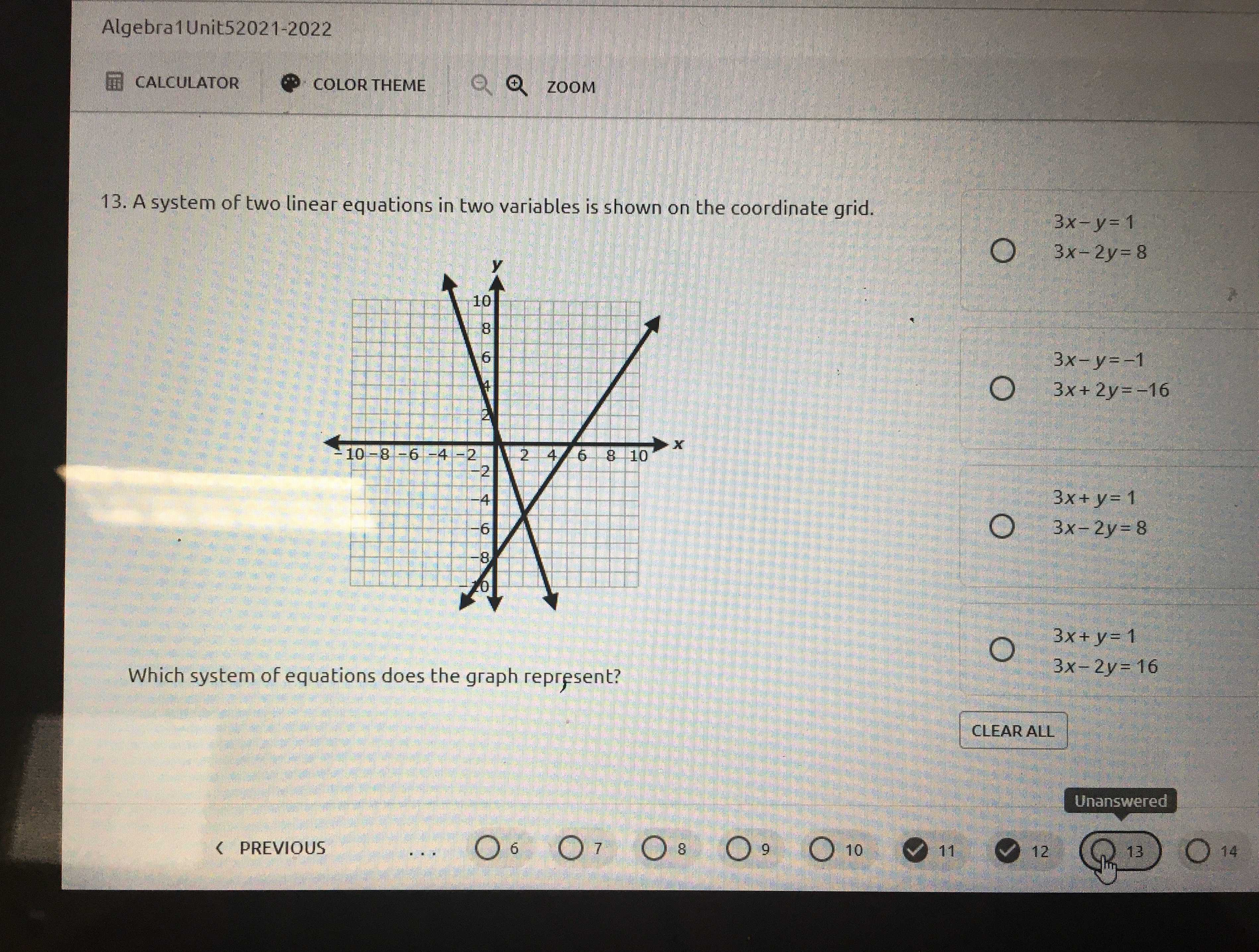### ¿Todavía tienes preguntas de matemáticas?

Pregunte a nuestros tutores expertos
Algebra
Pregunta13. A system of two linear equations in two variables is shown on the coordinate grid.

Which system of equations does the graph represent?

$$3 x - y = 1$$

$$3 x - 2 y = 8$$

$$3 x - y = - 1$$

$$3 x + 2 y = - 16$$

$$3 x + y = 1$$

$$3 x - 2 y = 8$$

$$3 x + y = 1$$

$$3 x - 2 y = 16$$

$$3 x + y = 1$$
$$3 x - 2 y = 16$$